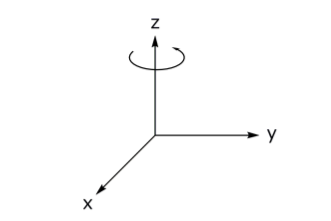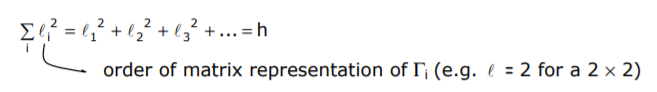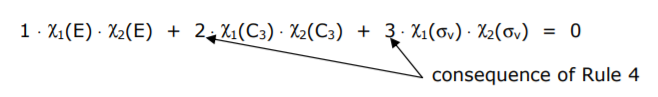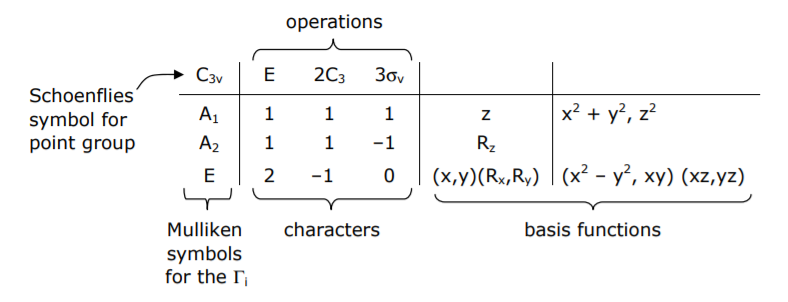# 1.3: Irreducible Representations and Character Tables

$$\newcommand{\vecs}{\overset { \rightharpoonup} {\mathbf{#1}} }$$ $$\newcommand{\vecd}{\overset{-\!-\!\rightharpoonup}{\vphantom{a}\smash {#1}}}$$$$\newcommand{\id}{\mathrm{id}}$$ $$\newcommand{\Span}{\mathrm{span}}$$ $$\newcommand{\kernel}{\mathrm{null}\,}$$ $$\newcommand{\range}{\mathrm{range}\,}$$ $$\newcommand{\RealPart}{\mathrm{Re}}$$ $$\newcommand{\ImaginaryPart}{\mathrm{Im}}$$ $$\newcommand{\Argument}{\mathrm{Arg}}$$ $$\newcommand{\norm}{\| #1 \|}$$ $$\newcommand{\inner}{\langle #1, #2 \rangle}$$ $$\newcommand{\Span}{\mathrm{span}}$$ $$\newcommand{\id}{\mathrm{id}}$$ $$\newcommand{\Span}{\mathrm{span}}$$ $$\newcommand{\kernel}{\mathrm{null}\,}$$ $$\newcommand{\range}{\mathrm{range}\,}$$ $$\newcommand{\RealPart}{\mathrm{Re}}$$ $$\newcommand{\ImaginaryPart}{\mathrm{Im}}$$ $$\newcommand{\Argument}{\mathrm{Arg}}$$ $$\newcommand{\norm}{\| #1 \|}$$ $$\newcommand{\inner}{\langle #1, #2 \rangle}$$ $$\newcommand{\Span}{\mathrm{span}}$$

Similarity transformations yield irreducible representations, Γi, which lead to the useful tool in group theory – the character table. The general strategy for determining Γi is as follows: A, B and C are matrix representations of symmetry operations of an arbitrary basis set (i.e., elements on which symmetry operations are performed). There is some similarity transform operator such that

$\begin{array}{l} \pmb A ^{\prime}=v^{-1} \cdot \pmb A \cdot v \\ \pmb B ^{\prime}=v^{-1} \cdot \pmb B \cdot v \\ \pmb C ^{\prime}=v^{-1} \cdot \pmb C \cdot v \end{array}$

where v uniquely produces block-diagonalized matrices, which are matrices possessing square arrays along the diagonal and zeros outside the blocks

\begin{equation}
\mathbf{A}^{\prime}=\left[\begin{array}{rrr}
\mathrm{A}_{1} & & \\
& \mathrm{~A}_{2} & \\
& & \mathrm{~A}_{3}
\mathrm{B}_{1} & & \\
& \mathrm{~B}_{2} & \\
& & \mathrm{~B}_{3}
\mathrm{C}_{1} & & \\
& \mathrm{C}_{2} & \\
& & \mathrm{C}_{3}
\end{array}\right]
\end{equation}

Matrices A, B, and C are reducible. Sub-matrices Ai, Bi and Ci obey the same multiplication properties as A, B and C. If application of the similarity transform does not further block-diagonalize A’, B’ and C’, then the blocks are irreducible representations. The character is the sum of the diagonal elements of Γi.

As an example, let’s continue with our exemplary group: E, C3, C3 2 , σv, σv’, σv” by defining an arbitrary basis … a triangleThe basis set is described by the triangles vertices, points A, B and C. The transformation properties of these points under the symmetry operations of the group are:

$E\left[\begin{array}{l} A \\ B \\ C \end{array}\right]=\left[\begin{array}{l} A \\ B \\ C \end{array}\right]=\left[\begin{array}{lll} 1 & 0 & 0 \\ 0 & 1 & 0 \\ 0 & 0 & 1 \end{array}\right]\left[\begin{array}{l} A \\ B \\ C \end{array}\right] \quad \sigma_{V}\left[\begin{array}{l} A \\ B \\ C \end{array}\right]=\left[\begin{array}{l} A \\ C \\ B \end{array}\right]=\left[\begin{array}{lll} 1 & 0 & 0 \\ 0 & 0 & 1 \\ 0 & 1 & 0 \end{array}\right]\left[\begin{array}{l} A \\ B \\ C \end{array}\right]$

$C _{3}\left[\begin{array}{l} A \\ B \\ C \end{array}\right]=\left[\begin{array}{l} B \\ C \\ A \end{array}\right]=\left[\begin{array}{lll} 0 & 1 & 0 \\ 0 & 0 & 1 \\ 1 & 0 & 0 \end{array}\right]\left[\begin{array}{l} A \\ B \\ C \end{array}\right] \quad \sigma_{ V }^{\prime}\left[\begin{array}{l} A \\ B \\ C \end{array}\right]=\left[\begin{array}{l} B \\ A \\ C \end{array}\right]=\left[\begin{array}{lll} 0 & 1 & 0 \\ 1 & 0 & 0 \\ 0 & 0 & 1 \end{array}\right]\left[\begin{array}{l} A \\ B \\ C \end{array}\right]$

$C _{3}^{2}\left[\begin{array}{l} A \\ B \\ C \end{array}\right]=\left[\begin{array}{l} C \\ A \\ B \end{array}\right]=\left[\begin{array}{lll} 0 & 0 & 1 \\ 1 & 0 & 0 \\ 0 & 1 & 0 \end{array}\right]\left[\begin{array}{l} A \\ B \\ C \end{array}\right] \quad \sigma_{ V }^{\prime \prime}\left[\begin{array}{l} A \\ B \\ C \end{array}\right]=\left[\begin{array}{l} C \\ B \\ A \end{array}\right]=\left[\begin{array}{lll} 0 & 0 & 1 \\ 0 & 1 & 0 \\ 1 & 0 & 0 \end{array}\right]\left[\begin{array}{l} A \\ B \\ C \end{array}\right]$

These matrices are not block-diagonalized, however a suitable similarity transformation will accomplish the task,

$$v=\left[\begin{array}{ccc} \frac{1}{\sqrt{3}} & \frac{2}{\sqrt{6}} & 0 \\ \frac{1}{\sqrt{3}} & -\frac{1}{\sqrt{6}} & \frac{1}{\sqrt{2}} \\ \frac{1}{\sqrt{3}} & -\frac{1}{\sqrt{6}} & -\frac{1}{\sqrt{2}} \end{array}\right] \quad ; \quad v^{-1}=\left[\begin{array}{ccc} \frac{1}{\sqrt{3}} & \frac{1}{\sqrt{3}} & \frac{1}{\sqrt{3}} \\ \frac{2}{\sqrt{6}} & -\frac{1}{\sqrt{6}} & -\frac{1}{\sqrt{6}} \\ 0 & \frac{1}{\sqrt{2}} & -\frac{1}{\sqrt{2}} \end{array}\right]$$

Applying the similarity transformation with C3 as the example,

$$v^{-1} \cdot \pmb C _{3} \cdot v=\left[\begin{array}{ccc} \frac{1}{\sqrt{3}} & \frac{1}{\sqrt{3}} & \frac{1}{\sqrt{3}} \\ \frac{2}{\sqrt{6}} & -\frac{1}{\sqrt{6}} & -\frac{1}{\sqrt{6}} \\ 0 & \frac{1}{\sqrt{2}} & -\frac{1}{\sqrt{2}} \end{array}\right] \cdot\left[\begin{array}{ccc} 0 & 1 & 0 \\ 0 & 0 & 1 \\ 1 & 0 & 0 \end{array}\right] \cdot\left[\begin{array}{ccc} \frac{1}{\sqrt{3}} & \frac{2}{\sqrt{6}} & 0 \\ \frac{1}{\sqrt{3}} & -\frac{1}{\sqrt{6}} & \frac{1}{\sqrt{2}} \\ \frac{1}{\sqrt{3}} & -\frac{1}{\sqrt{6}} & -\frac{1}{\sqrt{2}} \end{array}\right]$$

$$\left[\begin{array}{ccc} \frac{1}{\sqrt{3}} & \frac{1}{\sqrt{3}} & \frac{1}{\sqrt{3}} \\ \frac{2}{\sqrt{6}} & -\frac{1}{\sqrt{6}} & -\frac{1}{\sqrt{6}} \\ 0 & \frac{1}{\sqrt{2}} & -\frac{1}{\sqrt{2}} \end{array}\right] \cdot\left[\begin{array}{ccc} \frac{1}{\sqrt{3}} & -\frac{1}{\sqrt{6}} & \frac{1}{\sqrt{2}} \\ \frac{1}{\sqrt{3}} & -\frac{1}{\sqrt{6}} & -\frac{1}{\sqrt{2}} \\ \frac{1}{\sqrt{3}} & \frac{2}{\sqrt{6}} & 0 \end{array}\right]=\left[\begin{array}{ccc} 1 & 0 & 0 \\ 0 & -\frac{1}{2} & \frac{\sqrt{3}}{2} \\ 0 & -\frac{\sqrt{3}}{2} & -\frac{1}{2} \end{array}\right]= \pmb C _{3}^{*}$$

if v -1 C3*v is applied again, the matrix is not block diagonalized any further. The same diagonal sum is obtained *though off-diagonal elements may change). In this case, C3* is an irreducible representation, Γi.

The similarity transformation applied to other reducible representations yields:Thus a 3 × 3 reducible representation, Γred, has been decomposed under a similarity transformation into a 1 (1 × 1) and 1 (2 × 2) block-diagonalized irreducible representations, Γi. The traces (i.e. sum of diagonal matrix elements) of the Γi’s under each operation yield the characters (indicated by χ) of the representation. Taking the traces of each of the blocks:This collection of characters for a given irreducible representation, under the operations of a group is called a character table. As this example shows, from a completely arbitrary basis and a similarity transform, a character table is born.

The triangular basis set does not uncover all Γirr of the group defined by {E, C3, C3 2 , σv, σv’, σv’’}. A triangle represents Cartesian coordinate space (x,y,z) for which the Γis were determined. May choose other basis functions in an attempt to uncover other Γis. For instance, consider a rotation about the z-axis,The transformation properties of this basis function, Rz, under the operations of the group (will choose only 1 operation from each class, since characters of operators in a class are identical):

E: $$R_{z} \rightarrow R_{z}\ \quad C _{3}: R _{2} \rightarrow R _{2} \quad \sigma_{ v }( xy ): R _{2} \rightarrow \overline{ R }_{2}$$

Note, these transformation properties give rise to a Γi that is not contained in a triangular basis. A new (1 x 1) basis is obtained, Γ3, which describes the transform properties for Rz. A summary of the Γi for the group defined by E, C3, C3 2 , σv, σv’, σv” is:Is this character table complete? Irreducible representations and their characters obey certain algebraic relationships. From these 5 rules, we can ascertain whether this is a complete character table for these 6 symmetry operations.

Five important rules govern irreducible representations and their characters:

Rule 1

The sum of the squares of the dimensions, $$\ell$$, of irreducible representation Γi is equal to the order, h, of the group,Since the character under the identity operation is equal to the dimension of Γi (since E is always the unit matrix), the rule can be reformulated as,Rule 2

The sum of squares of the characters of irreducible representation Γi equals hRule 3

Vectors whose components are characters of two different irreducible representations are orthogonal

$$\sum_{R}\left[x_{i}(R)\right]\left[x_{j}(R)\right]=0 \quad$$ for $$\quad i \neq j$$

Rule 4

For a given representation, characters of all matrices belonging to operations in the same class are identical

Rule 5

The number of Γis of a group is equal to the number of classes in a group.

With these rules one can algebraically construct a character table. Returning to our example, let’s construct the character table in the absence of an arbitrary basis:

Rule 5: E (C3, C3 2 ) (σv, σv’, σv”) … 3 classes ∴ 3 Γis

Rule 1: $$\ell_{1}^{2}+\ell_{2}^{2}+\ell_{3}^{2}=6 \quad \therefore \ell_{1}=\ell_{2}=1, \ell_{2}=2$$

Rule 2: All character tables have a totally symmetric representation. Thus one of the irreducible representations, Γi, possesses the character set χ1(E) = 1, χ1(C3, C3 2 ) = 1, χ1v, σv’, σv”) = 1. Applying Rule 2, we find for the other irreducible representation of dimension 1,$$1 \cdot 1 \cdot x_{2}( E )+2 \cdot 1 \cdot x_{2}\left( C _{3}\right)+3 \cdot 1 \cdot x_{2}\left(\sigma_{ v }\right)=0$$

Since χ2(E) = 1,

$$1+2 \cdot x_{2}\left( C _{3}\right)+3 \cdot x_{2}\left(\sigma_{ v }\right)=0 \quad \therefore \quad \chi_{2}\left( C _{3}\right)=1, \chi_{2}\left(\sigma_{ v }\right)=-1$$

For the case of Γ3 ( $$\ell$$3 = 2) there is not a unique solution to Rule 2

$$2+2 \cdot \chi_{3} \left(C_{3}\right) +3 \cdot \chi_{3} \left(\sigma_{v}\right)=0$$

However, application of Rule 2 to Γ3 gives us one equation for two unknowns. Have several options to obtain a second independent equation:

Rule 1: $$1 \cdot 2^{2}+2\left[\chi_{3}\left(C_{3}\right)\right]^{2}+3\left[\chi_{3}\left(\sigma_{v}\right)\right]^{2}=6$$

Rule 3: $$1 \cdot 1 \cdot 2+2 \cdot 1 \cdot x_{3}\left(C_{3}\right)+3 \cdot 1 \cdot x_{3}\left(\sigma_{v}\right)=0$$
or
$$1 \cdot 1 \cdot 2+2 \cdot 1 \cdot x_{3}\left(C_{3}\right)+3 \cdot(-1) \cdot x_{3}\left(\sigma_{v}\right)=0$$

Solving simultaneously yields $$\chi_{3}\left(C_{3}\right)=-1, \chi_{3}\left(\sigma_{x}\right)=0$$

Thus the same result shown on pg 4 is obtained:

\begin{array}{c|ccc}
& \mathrm{E} & 2 \mathrm{C}_{3} & 3 \sigma_{\mathrm{v}} \\
\hline \Gamma_{1} & 1 & 1 & 1 \\
\Gamma_{2} & 2 & -1 & 0 \\
\Gamma_{3} & 1 & 1 & -1
\end{array}

Note, the derivation of the character table in this section is based solely on the properties of characters; the table was derived algebraically. The derivation on pg 4 was accomplished from first principles.

The complete character table is:• Γis of:

 $$\ell=1 \Longrightarrow A$$ or $$B$$ $$\ell=2 \Longrightarrow E$$ $$\ell=3 \Longrightarrow T$$ A is symmetric (+1) with respect to Cn B is antisymmetric (–1) with respect to Cn
• subscripts 1 and 2 designate Γis that are symmetric and antisymmetric, respectively to ⊥C2s; if ⊥C2s do not exist, then with respect to σv
• primes ( ’ ) and double primes ( ” ) attached to Γis that are symmetric and antisymmetric, respectively, to σh
• for groups containing i, g subscript attached to Γis that are symmetric to i whereas u subscript designates Γis that are antisymmetic to i

1.3: Irreducible Representations and Character Tables is shared under a CC BY-NC-SA 4.0 license and was authored, remixed, and/or curated by Daniel Nocera via source content that was edited to conform to the style and standards of the LibreTexts platform; a detailed edit history is available upon request.